# Grade 4 Order of Operations Practice

Students need to remember to follow the order of operations: parentheses first, then multiplication and division from left to right, and finally addition and subtraction from left to right.

Here's an example of a grade 4 order of operations practice:

5 x (4 + 2) - 8 =

First, you work on the equation between the parenthesis:

5 x 6 - 8 =

Then you work on the multiplication:

30 - 8 =

Finally, the subtraction:

30 - 8 = 22

In our grade 4 order of operations section, we have several sets of worksheets for students to solve equations involving the four operations with up to six terms and parentheses.

## Add and subtract with parentheses worksheets

Students work with five terms in these addition and subtraction worksheets. Students work with parentheses.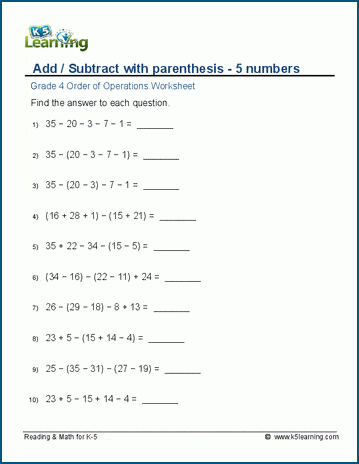## Add, subtract six terms with parentheses

This next set of worksheets has students add and subtract with six numbers and parentheses.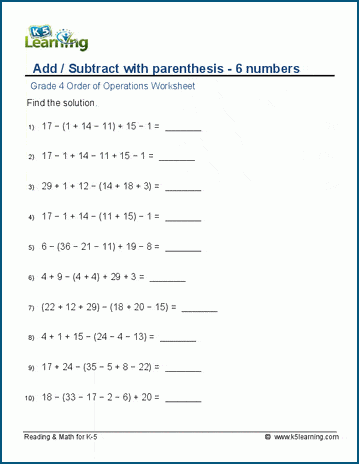## Practice adding, subtracting and multiplying in the correct order

These sets of worksheets introduce multiplication with addition and subtraction. Again, parentheses are in use.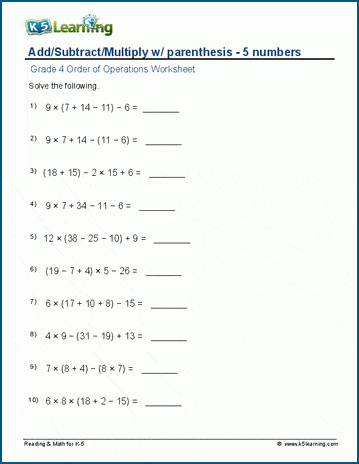## Adding, subtracting and multiplying six numbers

One more number is introduced in these worksheets, as students add, subtract and multiply 6 terms with parentheses.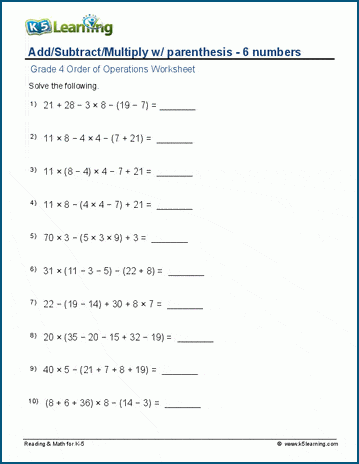## Add, subtract, multiply and divide equations in order of operations

Next, students are introduced to the four operations as they add, subtract, multiply and divide five numbers in the order of operations.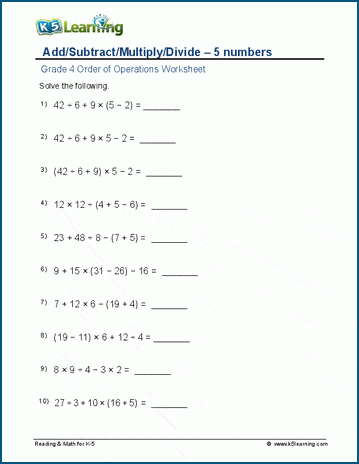## Add, subtract, multiply and divide six terms

Students work with 6 numbers in these, the most challenging, order of operations worksheets for grade 4 students.Become a Member

This content is available to members only.